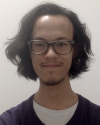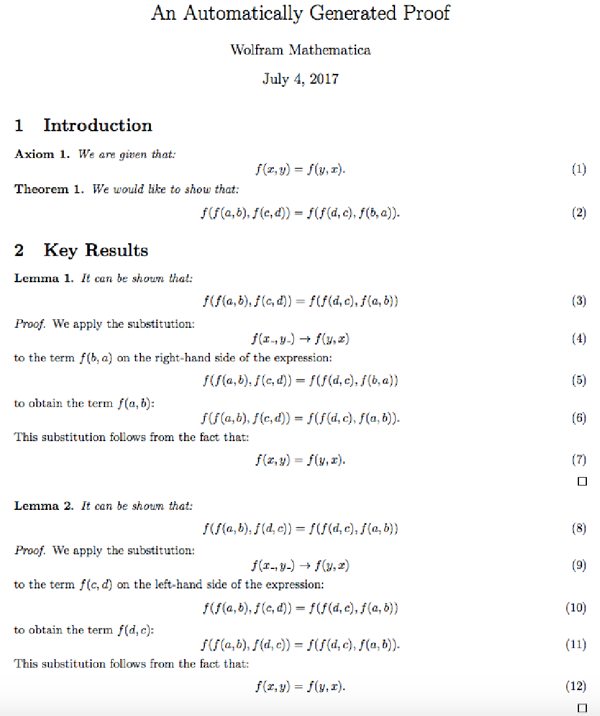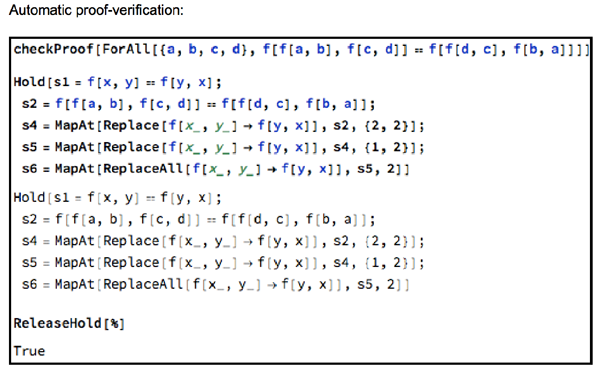#Wolfram Summer School

# Alumni# Jonathan Gorard

Science and Technology

Class of 2017

## Bio

Jonathan Gorard is a student and junior researcher in the department of mathematics at King’s College London, currently specializing in theoretical physics (in particular, the interface between cosmology and quantum field theory in the early universe). He also has deep interests in the areas of formal logic and computational complexity theory.

When he’s not busy doing nerdy things, Jonathan can be found going for long walks, reading and arguing about philosophy and attempting to play the guitar.

## Computational Essay

Monte Carlo Integration »

## Project: Automated Theorem Proving for Equational Logic

### Goal of the project:

To extend the back end of Mathematica’s FullSimplify function, in an effort to construct a system for automatically generating and visualizing proofs of arbitrary theorems in first-order equational logic.

### Summary of work:

I began by generalizing the preexisting Knuth–Bendix unfailing completion algorithm, thereby constructing a more sophisticated algorithm that would be able to auto-generate abstract proof networks of arbitrary theorems, not only in first-order equational logic but in any finite axiom system based on first-order equational logic. I subsequently experimented with methods for automatically presenting such proofs in human natural language, using various heuristics to determine the appropriate conceptual waypoints for guiding the reader through the logic of the proof. Finally, I implemented an automatic proof-verification algorithm, which is able to convert any such proof into executable Mathematica code.

### Results and future work:

With the framework for automatic proof generation, proof presentation and proof verification already implemented, the obvious next step would be to add functionality for exporting proofs in such a format that they could be uploaded directly to the Wolfram Data Repository. In addition, with further back end development, these tools could be used to gain deeper insight into certain longstanding results, such as the proof of correctness of the Wolfram axiom for Boolean algebra.## Wolfram Summer School | Champaign, IL, USA | July 3 29–July 22, 2022

APPLY NOW
• 21st Annual Wolfram Summer School
• Bentley University, Waltham, MA, USA
• June 25–July 15, 2023
• Apply now